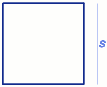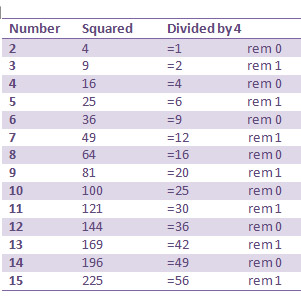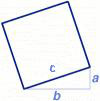#### You may also like### Some(?) of the Parts

A circle touches the lines OA, OB and AB where OA and OB are perpendicular. Show that the diameter of the circle is equal to the perimeter of the triangleA 1 metre cube has one face on the ground and one face against a wall. A 4 metre ladder leans against the wall and just touches the cube. How high is the top of the ladder above the ground?### At a Glance

The area of a regular pentagon looks about twice as a big as the pentangle star drawn within it. Is it?

# Grid Lockout

##### Age 14 to 16 Challenge Level:

Well done to Andrew (Wilson's School), Shibon (North London Collegiate School), and Jonathan (Oakwood Park Grammar School), who had the right answer to the situation when the squares are parallel to the lines on the grid.Shibon concluded that:

It is impossible to have a square whose area is (4n + 3) as all square numbers when divided by 4 have remainder of either 0 or 1 as shown below. No square number divided by 4 is left with a remainder of 3.Andrew sent us a good proof:

Let n be the integer we wish to square.
If n is even... let n = 2m
\begin{eqnarray} n^2 &=& 4m^2 \end{eqnarray}
Therefore n is divisible by 4.

If n is odd... let n = 2m+1
\begin{eqnarray} n^2 &=& (2m+1)^2 &=& 4m^2 + 4m + 1 &=& 4(m^2 + m) + 1 \end{eqnarray}
which must therefore be one more than a multiple of 4.

Therefore any integer, when squared, will leave a remainder of either 0 or 1 when divided by 4. Hence it is not possible to have a remainder 3.

For the situation when squares make angles with the lines of the grid, we have a good answer from Daniel (Savile Park).You can put right-angled triangles (sides a, b, and c) on any square.
Using Pythagoras' theorem: a² + b² = c²
So area of square = a² + b²

We have:
even number² = (2n)² = 4n² (which is divisible by 4)
odd number² = (2n + 1)² = 4n² + 4n + 1= 4(n² + n) + 1 (leaving a remainder of 1 when divided by 4)

Therefore:
even² + even² is divisible by 4
odd² + odd² leaves a remainder of 2 when divided by 4
even² + odd² leaves a remainder 1 when divided by 4

So a² + b² never has a remainder of 3 when divided by 4.
Hence the area of a square can't be 4n + 3